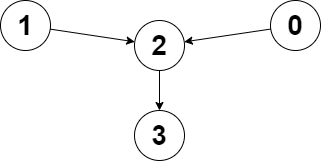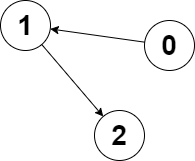# 2359. Find Closest Node to Given Two Nodes Solution

You are given a directed graph of `n` nodes numbered from `0` to `n - 1`, where each node has at most one outgoing edge.

The graph is represented with a given 0-indexed array `edges` of size `n`, indicating that there is a directed edge from node `i` to node `edges[i]`. If there is no outgoing edge from `i`, then `edges[i] == -1`.

You are also given two integers `node1` and `node2`.

Return the index of the node that can be reached from both `node1` and `node2`, such that the maximum between the distance from `node1` to that node, and from `node2` to that node is minimized. If there are multiple answers, return the node with the smallest index, and if no possible answer exists, return `-1`.

Note that `edges` may contain cycles.

Example 1:```Input: edges = [2,2,3,-1], node1 = 0, node2 = 1
Output: 2
Explanation: The distance from node 0 to node 2 is 1, and the distance from node 1 to node 2 is 1.
The maximum of those two distances is 1. It can be proven that we cannot get a node with a smaller maximum distance than 1, so we return node 2.
```

Example 2:```Input: edges = [1,2,-1], node1 = 0, node2 = 2
Output: 2
Explanation: The distance from node 0 to node 2 is 2, and the distance from node 2 to itself is 0.
The maximum of those two distances is 2. It can be proven that we cannot get a node with a smaller maximum distance than 2, so we return node 2.
```

Constraints:

• `n == edges.length`
• `2 <= n <= 105`
• `-1 <= edges[i] < n`
• `edges[i] != i`
• `0 <= node1, node2 < n`

## Solution-

We will store the path from both nodes and then will take out the common points in them. Now we will see the distances from both the nodes and if it satisfies the given condition then we will update our answer.

class Solution {
public:

void helper(vector<int>&v,int node,vector<bool>&vis, unordered_map<int,int>&m, int dis){
vis[node]=true;
m[node]=dis;
if(v[node]!=-1 && !vis[v[node]])helper(v,v[node],vis,m,dis+1);
}

int closestMeetingNode(vector<int>& edges, int node1, int node2) {
vector<bool>vis(edges.size()+1,false);
unordered_map<int,int>m1,m2;
helper(edges,node1,vis,m1,0);
for(int i=0; i<vis.size(); i++)vis[i]=false;
helper(edges,node2,vis,m2,0);
int ans=-1,dis=INT_MAX;
for(auto i:m1){
auto it=m2.find(i.first);
if(it==m2.end())continue;
int mx=max(i.second,it->second);
if(mx<dis){
ans=i.first;
dis=mx;
}
else if(mx==dis)ans=min(ans,i.first);
}
return ans;
}
};